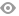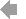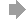Basic HTML VersionView Full Version
Page 157 - University of Pretoria RESEARCH OUTPUTS 2016
P. 157

``````Akimenko V, and Anguelov R. 2016. Steady states and outbreaks of two-phase nonlinear age-structured model of population dynamics with discrete time delay. In Journal of Biological Dynamics, 11(1), pp 75-101. http://hdl.handle.net/2263/58617.
Ali B, Abbas M, and Costache S. 2016. Fixed fuzzy point theorems for fuzzy mappings on complete metric spaces. In UPB Scienti c Bulletin, Series A: Applied Mathematics and Physics, 78, pp 31-42.
Ali ZI, Sango M. 2016. A note on weak and strong probabilistic solutions for a stochastic quasilinear parabolic equation of generalized polytropic  ltration. In International Journal Of Modern Physics B, 30, pp 1640002-1640013.
Ali ZI, Sango M. 2016. Weak and Strong Probabilistic Solutions for a Stochastic Quasilinear Parabolic Equation with Nonstandard Growth. In Russian Journal of Mathematical Physics, 23, pp 283-308.
Appadu AR, Djoko Kamdem J, and Gidey HH. 2016. A computational study of three numerical methods for some advection-di usion problems. In Applied Mathematics and Computation, 272, pp 629-647.
Baker C, Rajaratnam K, and Flint EJ. 2016. Beta estimates of shares on the JSE Top 40 in the context of reference-day risk. In Environment Systems & Decisions, 36, pp 126-141. http://hdl.handle.net/2263/59463.
Banasiak J. 2016. Explicit formulae for limit periodic  ows on networks. In Linear Algebra and its Applications, 500, pp 30-42. Banda MK, Hack A, and Herty M. 2016. Numerical Discretization of Coupling Conditions by High-Order Schemes. In Journal of
Scienti c Computing, 69, pp 122-145. http://hdl.handle.net/2263/56342.
Borowiecki M, and Broere I. 2016. Hamiltonicity and generalised total colourings of planar graphs. In Discussiones Mathematicae
Graph Theory, 36, pp 243-257. http://hdl.handle.net/2263/56042.
Broere I, Matsoha MDV, and Heidema J. 2016. The quest for characterization of hom-properties of  nite character. In Discussiones
Mathematicae Graph Theory, 36, pp 479-500.
Chapwanya M, Lubuma JM, and Terefe YA. 2016. Analysis and dynamically consistent nonstandard discretization for a rabies model in humans and dogs. In Revista de la Real Academia de Ciencias Exactas Fisicas y Naturales Serie A-Matematicas, 110, pp 783-798.
Ciric L, Chandok S, and Abbas M. 2016. Invariant approximation results of generalized contractive mappings. In Filomat, 30(14), pp 3875-3883. http://hdl.handle.net/2263/59088.
Clarkson P, Jordaan KH, and Kelil AS. 2016. A Generalized Freud Weight. In Studies in Applied Mathematics, 136, pp 288-320.
http://hdl.handle.net/2263/55754.
De la Sen M, Abbas M, and Saleem N. 2016. On optimal fuzzy best proximity coincidence points of fuzzy order preserving proximal Ψ(σ, α)‐lower‐bounding asymptotically contractive mappings in non‐Archimedean fuzzy metric spaces. In SpringerPlus, 5(1478),
pp 1-26.
Djoko Kamdem J, and Koko J. 2016. Numerical methods for the Stokes and Navier–Stokes equations driven by threshold slip boundary conditions. In Computer Methods in Applied Mechanics and Engineering, 305, pp 936-958.
Djoko Kamdem J, Lubuma JM, and Mbehou M. 2016. On the Numerical Solution of the Stationary Power-Law Stokes Equations: A Penalty Finite Element Approach. In Journal of Scienti c Computing, 69, pp 1058-1082.
Djoko Kamdem J, Lubuma JM. 2016. Analysis of a Time Implicit Scheme for the Oseen Model Driven by Nonlinear Slip Boundary Conditions. In Journal of Mathematical Fluid Mechanics, 18, pp 717-730.
Djouosseu Tenkam HM, Anguelov R, Fedotov I, and Shatalov M. 2016. Exact solution of the Mindlin–Herrmann model for longitudinal vibration of an isotropic rod. In Journal of Engineering Mathematics, 99, pp 185-201. http://hdl.handle.net/2263/50923.
Djouosseu Tenkam HM, Shatalov M, Fedotov I, and Anguelov R. 2016. Mathematical Models for the Propagation of Stress Waves in Elastic Rods: Exact Solutions and Numerical Simulation. In Advances in Applied Mathematics and Mechanics, 8, pp 257-270.
UP Research Outputs 2016 | 155

``````155   156   157   158   159